# The area between two curves calculator – Method and Applications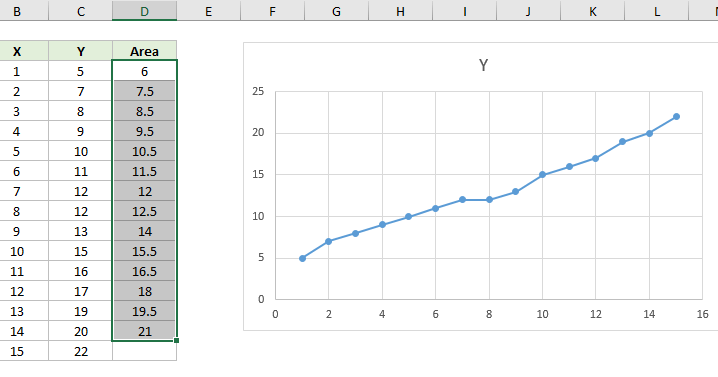In the context of Mathematics, we have often used integral calculus to find the area under a curve. Similarly, it is also true that we can find the area between two curves. To do this, we apply the concepts of definite integrals. However, the area between two curves calculator will help you in performing these calculations in a much easier way.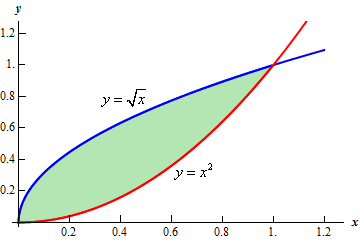### The area between two curves formula:

As we have said earlier, we will use the concepts of definite integrals to find the area between two curves. Besides being an important application of definite integrals, these problems also give one an idea about the nature of the two curves involved.

So, the formula for calculating the area between two curves looks like this:

A = [f(x)–g(x)]dx,

Where f(x) is the equation of one curve and g(x) is the equation of the other curve and,

x1 and x2 are the bounding values of x (in this case).

### The area between two curves calculator:

The area between two curves calculator is an online tool that effectively calculates the area between two curves.

Since finding the area between two curves is a crucial application of integral calculus, we can use this calculator for a lot of reasons.

• The calculator provides very accurate results.
• Also, the area between two curves calculator is pretty easily available.
• Additionally, it is not at all time-consuming, as compared to the

pen-and-paper-based method.

• Also, you do not need to know the area between curves

formula for using the area between two curves calculator.

### The area between two curves calculator with steps:

It is quite easy to calculate the area between two curves when we know the points of their intersection. Also, the points of intersection of the two curves are called the lower and the upper bounds.

In conclusion, it exists between two points, it is calculated by computing the definite integral between the two points.

The calculation for area between two curves

y= f(x) between x= a & x= b

y= f(x) between limits of a & b ( b should be greater than a).

Follow these steps to obtain correct results.

• Firstly, write the first function in the space provided to you. Actually, this is the equation of the first curve.
• Secondly, write the second function in the space provided to you. Actually, this is the equation of the second curve.
• Thirdly, put in the left bound and the right bound of the functions. The left and the right bounds are nothing but the points of intersection of the two curves.
• Finally, click on the ‘Submit’ button. The output box will display the result accordingly.

There are a few conventions that you need to keep in mind.

They are:

• The upper curve / function in a graph is f(x).
• The lower curve / function in a graph is g(x).
• We will assign positive signs to the area above the y-axis.
• We will assign negative signs to the area below the y-axis.

### Area of two curves using integration:

The area between two curves is calculated using integration. Now, we will explain the underlying concept of this application. Actually, the area that lies in the intersection area of the two curves is divided into thin rectangular strips.

Now, add the individual areas of these thin stripes to find the combined area of this region.

Suppose, the width of these rectangular strips is ‘dx’.

Thus, calculate the area of this zone as  A =[f(x)–g(x)]dxSimilarly, the area between two curves can also be found along the y-axis.

However, we will discuss this in the next section.

### Area of two curves calculator with respect to y-axis:

You can also find the area between two curves along the y-axis.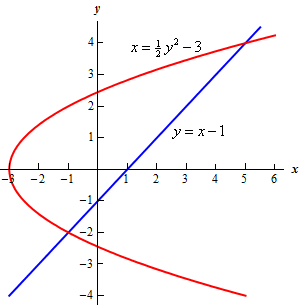In fact, this is easier to find.

When the equation of a curve is expressed in terms of y, the area

between the curves can be calculated along the y-axis.

Now, divide the area within the limits into thin horizontal stripes. In this

case, add the areas of the horizontal stripes to calculate the total area

of this region.

Suppose, f(y) and g(y) are continuous on [c,d], then

A = [f(y)–g(y)] dy.

### The area between the two curves polar

A polar curve is a shape constructed using the polar coordinate system. Polar curves are defined by points that are a variable distance from the origin (the pole) depending on the angle measured off the positive x-axis. There are five classic polar curves: cardioids, limaҫons, lemniscates, rose curves, and Archimedes’ spirals.However, follow these steps to find the area between two polar curves:

Firstly, find the points of the intersection if you do not know the interval.

Secondly, graphing the curves is also quite important. This confirms the exact Intervals.

Thirdly, For each enclosed region, use the points of intersection to find upper and lower limits of integration [α,β]

Fourthly, for any enclosed region, specify the outer curve and the

inner curve.

Finally, plug this into the formula for the area between curves,

A =  1 / 2​​ (ro^2 – rI^2) dθ

where [α,β] is the interval that defines the area,

where​​ ro is the outer curve, and,

where​​ rI is the inner curve.### The area between two curves calculator desmos

The area between two curves calculator (polar) is also available online very easily.

Actually, you do not have to remember the formula for calculating the area between two polar curves. So, this calculation becomes a lot easier.

Firstly, plug in the outer curve’s equation in the f(θ) box. Function f is the blue curve.

Secondly, plug in the inner curve’s equation in the g(θ) box.

Function g is the green curve.

Further, The equation gives us the area between two curves:

(n1 / 2)  f(θ)^2 dθ + (n2 / 2)  g(θ)^2 dθ

From the graph, n1 is the number of green sections needed to complete or negate to achieve the desired area.

Further, n2 is the number of blue sections needed to complete or negate in order to achieve the desired area. Thus, after you put in this information, the output box displays the result.

### The area between two curves calculator symbolab

This is yet another online calculator that is used to find the area between two curves. This calculator is basically an easy way to integrate functions. In fact, all you need to do is choose the integration sign. Then, you can write the required function in the provided box.

Finally, the answer is automatically displayed.

Also, we are providing you the link to access this calculator:

calculator symbolab

### The area between two curves calculator mathway

This calculator by Mathway will help you to find the area between two curves in just a fraction of a few seconds.

Firstly, consider two equations for the two curves.

Initially, solve by substitution to find the intersection between the curves.

Then, Eliminate the equal sides of each equation and combine.

The solution to the system is the complete set of ordered pairs that are valid solutions.

The area of the region between the curves is defined as the integral of the upper curve minus the integral of the lower curve over each region. The regions are determined by the intersection points of the curves.

However, this can be done algebraically or graphically.

You can enter any problem you want in the space provided. This calculator will then display the result along with a detailed explanation of all the included steps.

Here, we are providing you the link to access this calculator by Mathway:

mathway

### Volume of area between two curves calculator:

By now, you know how you can easily calculate the area between two curves. Now, we can proceed to find the volume of the area between two curves, as well.

For calculating this, you can easily use the ‘volume of area between two curves’ calculator. This calculator is available online. You just need to plug in the equations of the two curves and the boundary points that bound the region between them. Then, the answer is automatically displayed.

Further, this calculator is also called the Volume of solid of revolution Calculator.

We are also attaching the link for this Volume of solid of revolution Calculator presented by symbolab:

### Example 1:

Find the area between the curves y=0 and y = 3 ( – x).

When we graph the region, we see that the curves cross each other so that the top and bottom switch. Hence we split the integral into two integrals:

Thus, the graph now looks like this:[3 ( – x) – 0] dx +  [0 – 3 ( – x)] dx

Or, (3 / 4 *  – 3 / 2 ) integrated over [-1 to 0] – (3 / 4 *  – 3 / 2 ) integrated over [0 to 1].

Or, (- 3 / 4 + 3 / 2 ) – (3 / 4 – 3 / 2 ) = 3 / 2.

Thus, the area between these two curves is 3 / 2 units.

### Example 2:

Find the area between the curves x = 1 −  and x =− 1.

Here the curves bound the region from the left and the right.

The graph for these two curves will look as follows: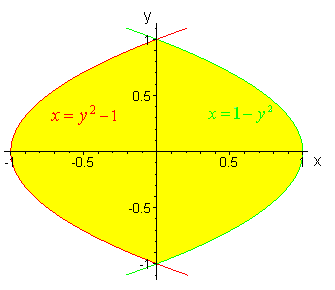Here, we use the formula:

Area =  (Right – Left) dy.

For our example,

[ (1 − y^2) − (y^2 − 1) ] dy

Or, (2 – y^2) dy

Or, [ 2 y – (2 / 3)y^3] over the definite interval -1 to +1.

Or, [2 – (2 / 3)] – [- 2 – (2 / 3)]  = 8 / 3.

Thus, the area between the two curves is 8 / 3 units.

### Example 3:

Let us now use this concept in the context of solving an economic problem.

Let y=f(x) be the demand function for a product and y=g(x) be the supply function. Then, we define the equilibrium point to be the intersection of the two curves. The consumer surplus is defined by the area above the equilibrium value and below the demand curve, while the producer surplus is defined by the area below the equilibrium value and above the supply curve.

Find the producer surplus for the demand curve f(x) = 1000 – 0.4 x^2 and the supply curve g(x) = 42 x.

Actually, the graph can be drawn as follows: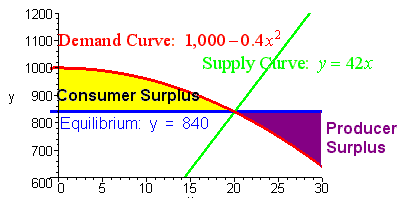Further, we first find the equilibrium point:

We set f(x) = g(x) [Since the demand and supply must be the same at equilibrium]

Thus, write it as:

1000 – 0.4 x^2 = 42 x

Or,  0.4 x^2 + 42 x – 1000 = 0.

So, we get x = 20.

Hence, y = 42 * 20 = 840.

So, you can now integrate:

(840 – 42x) dx

Or, [840 – 21 x*2] over the interval 0 to 20.

Or, 8400.

Here are a few other problems for you to practice:

1. Find the area between the curves y = x^2 and y = (x)^½.
2. Calculate the area between the curves y = x^2 – 4 and y = – 2x.
3. Compute the area between the curves y = 2 / x and y = -x + 3.
4. Find the area between the curves y = x*(3)^x and y = 2x +3.

### The area between two curves applications real life:

#### 1.   Lorenz curve:

The Lorenz Curve L(x) gives the proportion of the total income earned by the lowest proportion x of the population. It can also be used to show the distribution of assets (total wealth, rather than income). Economists consider it to be a measure of social inequality.

However, Max O. Lorenz developed it in 1905 to represent the inequality of wealth distribution.From this figure,

A Lorenz curve L(x) compared to the diagonal line which represents perfect income equality. Region A represents the difference between perfect income equality and actual income distribution. Experimentally, the larger A is, the more unequal the income distribution is.

#### 2.   Consumer Surplus and Producer Surplus:Consumer surplus is the difference between the total amount that consumers are willing and able to pay for a good or service (indicated by the demand curve) and the total amount that they actually do pay (i.e., the actual market price for the product). Thus, the level of consumer surplus is shown by the area below the demand curve and above the ruling market price line as illustrated.

On the contrary, producer surplus is the difference between the total amount that producers of a good receive and the minimum amount that they would be willing to accept for the good (blue shading).

Thus, the concept of the area under curves/area between two curves is important for the consumer and the producer surplus calculations.

### The area between two curves using EXCEL: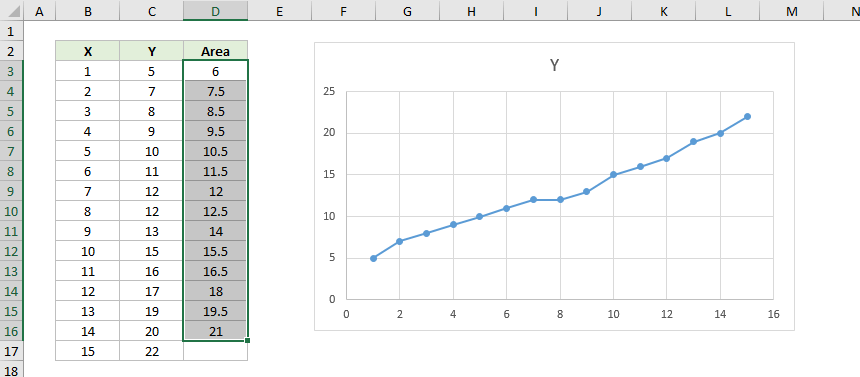Follow these steps to find the area between two curves using EXCEL:

1. Type “LINEST(yrange, xrange, TRUE, FALSE)” into a blank cell in your Excel spreadsheet.
2. Change the options in the LINEST command to reflect the location of your data for one of the graphs. For example, if your y-data is in cells A1:A8 and your x-data is in cells B1:B8 then the function would read LINEST(A1:A8, B1:B8, TRUE, FALSE).
3. Press “Enter” to get the equation for the first set of data.
4. Type “LINEST(yrange, xrange, TRUE, FALSE)” into a second blank cell in your Excel spreadsheet.
5. Change the options in the LINEST command to reflect the location of your actual data for the second graph. For example, if the y-data for your second graph is in cells C1:C8 and your x-data is in cells D1:D8 then the function would read LINEST(C1:C8, D1:D8, TRUE, FALSE).
6. Now, press “Enter” to get the equation for the second set of data.
7. Next, calculate the definite integral for the two equations. You will require a knowledge of calculus for this part. There are online calculators available to calculate definite integrals. For example, many sites offer online definite integral calculators. We have also provided one in our article.
8. Calculate the area under the first curve using the definite integral.
9. Calculate the area under the second curve using the definite integral.
10. Subtract Step 9 from Step 8 to find the area between the two curves.

### The area between two curves calculator FAQs

#### 1.   Can the area between two curves be negative when we use the calculator?

Ans: No, the result obtained from the area between two curves calculator can never be negative. However, the typical method of solution in that instance is to consider each piece separately, integrating (top function) – (bottom function) for each piece, to guarantee a positive (nonnegative) result. By definition, “Area between two graphs” is positive regardless of where in the plane it lies.

#### 2.   What does the area between the two curves’ calculator’s output signify?

Ans: The value obtained from the area between two curves calculator gives us the area of the total region occupied between the two curves in the coordinate plane.

#### 3.   What is the concept behind finding the area between two curves?

Ans: The area under a curve between two points can be found by doing a definite integral between the two points. Thus, to find the area under the curve y = f(x) between x = a and x = b, integrate y = f(x) between the limits of a and b.

#### 4.   How do you find the area between two curves without graphing?

Ans: If you do not want to use the graphing method to find the area between two curves, you can simply put the function into the area between the two curves calculator. The basic idea is to compute the definite integral from a to b. You have to find the absolute value of the function at ‘a’ and subtract it from the absolute value of the function at ‘b’.

#### 5.   What is the first step towards finding the area between two curves?

Ans: Whenever you need to find the area between two curves, the first step you should consider to solve the problem is to graph it. Also, this makes the solving process a lot easier.

When you graph the required curves properly, the point of intersection becomes easier to find. Even, the shape of the region in the middle and the boundary points which bound this region also become clear. So, in total, the entire process becomes very easy and you can calculate the area between the two given curves without facing any difficulty at all.

#### 6.   Is it possible to get the area between y2 = 3x and y = x3 using: horizontal strips or vertical strips?

Ans: Yes, it is possible to get the area between these two functions either along the horizontal axis or along the vertical axis. Firstly, we have to draw the graph for the two curves. Then, divide the region between the two curves into either thin horizontal strips or thin vertical strips. Finally, you can proceed using the process that we have illustrated in our article above.